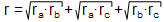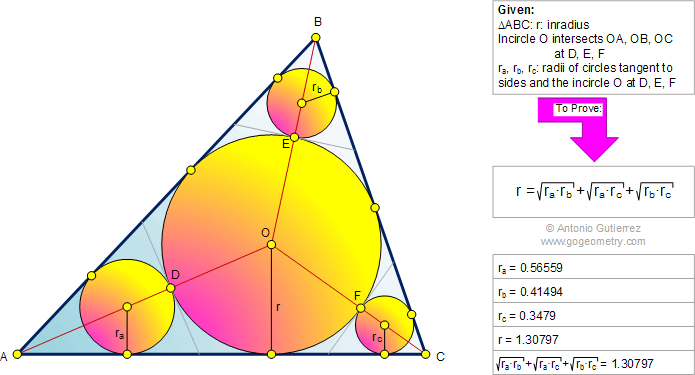# Online Geometry Problem 454: Triangle, Incircle, Inradius, Tangent, Circle, Radius, Radii. Level: High School, Honors Geometry, College, Mathematics Education

 The figure shows a triangle ABC with the incircle O of radius r. Circle O intersects OA, OB and OC at D, E, and F, respectively. If ra, rb, and rc, are the radii of circles tangent to the sides and to the incircle O at D, E, and F, respectively, prove that.Home | Search | Geometry | Problems | All Problems | Open Problems | Visual Index | 451-460 | Triangles | Circles | Tangent Circles | Incircle | Circle Tangent Line | Angle Bisector | Problem 1095 | Email | Solution / comment | By Antonio GutierrezLast updated: Mar 10, 2015